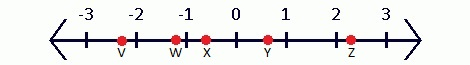# Comparing and ordering rational numbers

## Understanding the number systems

When people talk about number systems, what are they referring to? Number systems are ways to represent numbers. The most common number system is the decimal system, which has a base of 10. This is the one we use most frequently in our daily lives.

Within number systems, there are rational and irrational numbers. Rational numbers are numbers that can be expressed as simple fractions. This includes whole numbers and natural numbers that you use in counting. Numbers with terminating decimals such as 1.75 is rational because it can also be written as $\frac{7}{4}$ in fraction form. You can even have a number with repeating decimals as a rational number since it can be expressed as a fraction. On the other hand, Pi ($\pi$) is one of the more famous irrational numbers. As you probably know, $\pi$ is also known as 3.14159265… and this chain of number continues. There are no simple fractions that can express that number.

Now that you know what a rational number is, let's move on to learning how to put these numbers in order.

## Ordering rational number

One of the most basic lessons in math is to compare and order numbers. So how do we put rational numbers into ascending or descending order? You can represent them on a number line just like integers! You can put negative and positive rational numbers in order, but one thing to do first is to convert them to the same form. If some of your numbers are in fractions while others are in decimals, you should change them all to decimals before proceeding so that you don't get confused. It's much simpler to order rational numbers when they're in the same form.

To do a quick review of the greater than and less than symbols, > stands for greater than, and < stands for smaller than. Numbers are placed on the left and right sides of this symbol to show which number is bigger or smaller. You'll need to remember this as you tackle questions in this lesson.

## Practice problems

Question 1

Matching the rational number to a point on the number line.

• a) $\frac{3}{7}$
• b) -$1 \frac{1}{5}$
• c) -$2 \frac{1}{4}$
• d) $2 \frac{5}{18}$
• e) -$0.6$

Solution:

First, let's convert the fractions so that they're all decimals:

• a) 0.43
• b) -1.2
• c) -2.25
• d) 2.28
• e) -0.6

Now that they're more easily comparable, we can estimate where they would lie on the above number line. All we'll have to do is match the points:

• a) Y
• b) W
• c) V
• d) Z
• e) X

Question 2:

Compare $-\frac{1}{6},-1\frac{3}{4}, 1\frac{5}{7}, -0.2, 2.1$. Write the numbers in descending order.

Solution:

• Again, let us first convert each number to its decimal form first.
• We'll get: -0.17, -1.75, 1.71, -0.2, 2.1.
• Then, arrange your numbers in descending order:
• 2.1 > 1.71 > -0.17 > -0.2 > -1.75
• Make sure to count the number of numbers in your final answer to make sure you've included all of them when doing the ordering.

Question 3:

Which value in the following pair is smaller?

$\frac{2}{5}, -\frac{3}{5}$

Solution:

• In decimal form, we get the following numbers from the above fractions:
• 0.4, -0.6
• -0.6 is smaller.

If you needed more practice questions on comparing and ordering rational numbers, here's a worksheet for you to try out!

### Comparing and ordering rational numbers

A rational number is a number that can be written as a fraction. In order to be called a rational number, the numerator and denominators of the fraction must be whole numbers. In this lesson, we will compare different rational numbers and sort out their order.

#### Lessons

• 1.
Match the rational number to a point on the number line.a) $\frac{3}{7}$
b) -1$\frac{1}{5}$
c) -2$\frac{1}{4}$
d) 2$\frac{5}{{18}}$
e) -0.6

• 2.
Compare -$\frac{1}{6}$, -1$\frac{3}{4}$, 1$\frac{5}{7}$, -0.2, and 2.1. Write the numbers in descending order.

• 3.
Which value in each pair is smaller?
a)
$\frac{2}{5}$, -$\frac{3}{5}$

b)
-2$\frac{1}{3}$, -2$\frac{1}{6}$

c)
-$\frac{{13}}{{25}}$, $\frac{{11}}{{25}}$

d)
-$\frac{1}{3}$, -$\frac{4}{7}$

• 4.
Find a mixed number between each pair of rational numbers below.
a)
-0.7, 1.7

b)
3.5, 3.6

c)
-4.02, -4.04

d)
-2.8, -2.9